Distributing mobs in a dungeon based on player’s level (or some dungeon level difficulty factor) was somewhat straightforward, but I would still like to document the progress. I needed to place a mob that’s somewhat within the difficulty level I want, plus minus a few difficulty levels to spice it up.Above you can see three rats, three cats, a dog (`r`, `c`, `d`, all level 1), a farmer (`f`, level 2), and a lonely bandit (`b`, level 3) in a level 1 dungeon.

Without going straight into measure theory, I generated intervals for each mob based on the diff of desired level and their level, and then randomly selected a point within the boundaries. Here’s the abstract code:

``````import bisect
import random

def get_random_element(data, target, chance):
"""Get random element from data set skewing towards target.

Args:
data   -- A dictionary with keys as elements and values as weights.
Duplicates are allowed.
target -- Target weight, results will be skewed towards target
weight.
chance -- A float 0..1, a factor by which chance of picking adjacent
elements decreases (i.e, with chance 0 we will always
select target element, with chance 0.5 probability of
selecting elements adjacent to target are halved with each
step).

Returns:
A random key from data with distribution respective of the target
weight.
"""
intervals = []  # We insert in order, no overlaps.
next_i = 0
for element, v in data.iteritems():
d = max(target, v) - min(target, v)
size = 100
while d > 0:  # Decrease chunk size for each step of `d`.
size *= chance
d -= 1
if size == 0:
continue
size = int(size)
intervals.append((next_i, next_i + size, element))
next_i += size + 1
fst, _, _ = zip(*intervals)
rnd = random.randint(0, next_i - 1)
idx = bisect.bisect(fst, rnd)  # This part is O(log n).
return intervals[idx - 1]
``````

Now, if I test the above for, say, a 1000000 iterations, with a chance of 0.5 (halving probability of selecting adjacent elements with each step), and 2 as a target, here’s the distribution I end up with:

``````target, chance, iterations = 2, 0.5, 1000000

data = collections.OrderedDict([  # Ordered to make histogram prettier.
('A', 0), ('B-0', 1), ('B-1', 1), ('C', 2), ('D', 3), ('E', 4),
('F', 5), ('G', 6), ('H', 7), ('I', 8), ('J', 9),
])

res = collections.OrderedDict([(k, 0) for k, _ in data.iteritems()])

# This is just a test, so there's no need to optimize this for now.
for _ in xrange(iterations):
res[get_random_element(data, target, chance)] += 1

pyplot.bar(
range(len(res)), res.values(), width=0.7, align='center', color='green')
pyplot.xticks(range(len(res)), res.keys())
pyplot.title(
'iterations={}, target={}, chance={}'.format(
iterations, target, chance))
pyplot.show()
``````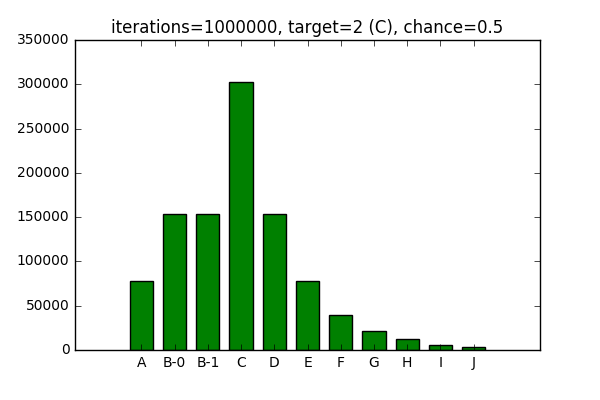You can see elements B-0 and B-1 having roughly the same distribution, since they have the same weight.

Now, if I decrease the `chance`, likelihood of target being selected increases, while likelihood of surrounding elements being selected decreases: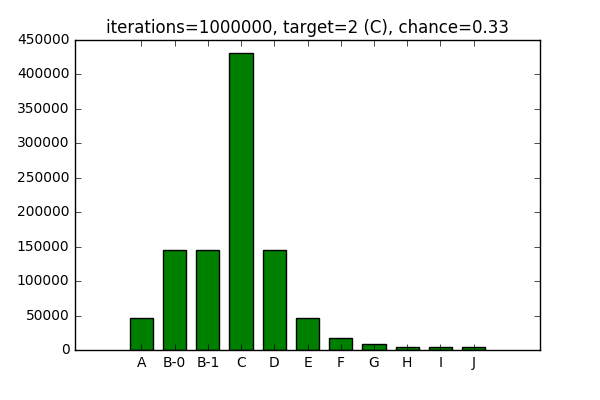I works the opposite way as well, increasing the `chance` decreases likelihood of the target being selected and increases the probability for surrounding elements.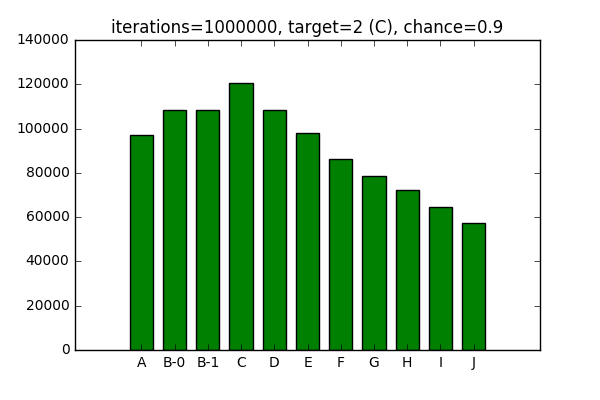For the sake of completeness, it works with 0 chance of surrounding elements being picked: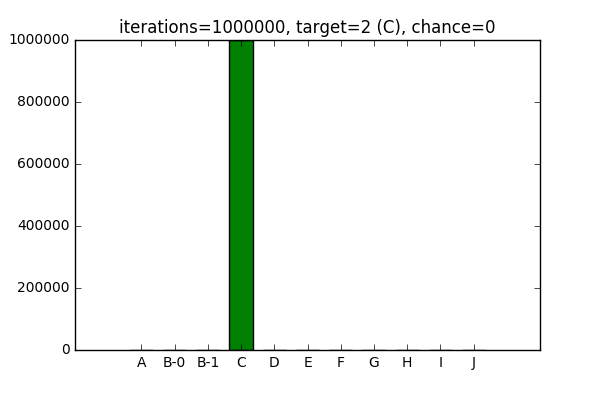And an equal chance of picking surrounding elements: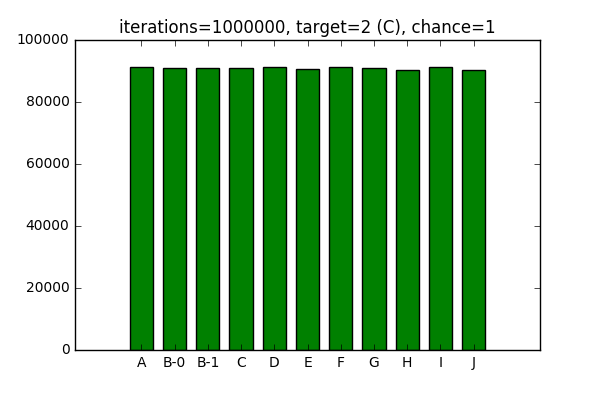After playing around with the configuration in Jupyter Notebook, I cleaned up the algorithm above and included it into mob placement routine.